# ML Wiki

## Sparse Index

A sparse index has one (key, pointer) per each block

• so it uses less space than Dense Index
• but requires more time to find a record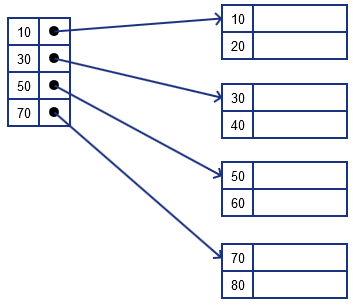• not all keys are referenced by this index
• pointers point only to the 1st key of the block
• cannot say from index if a key is present or not, always need to follow a pointer, load the block and check the key there

### Nested Levels

Key benefit of this

• we can build the 2nd index on top of index
•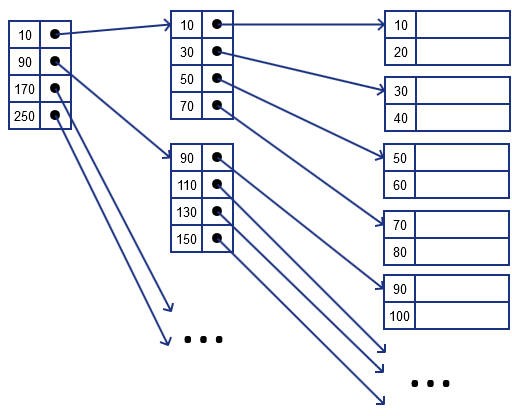## Operations

### Lookup

Rule to retrieve record with specified $k$

• we find such $k$ in index that
• is greater or equal to $k$,
• but less than consequent one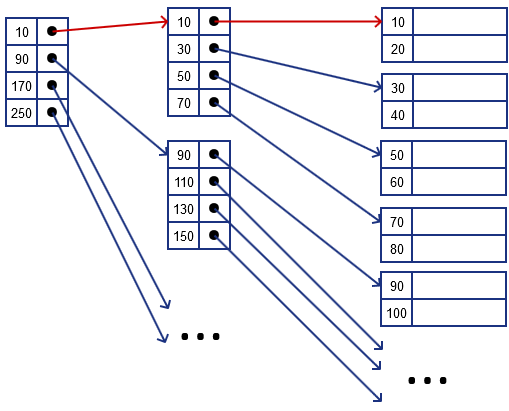• suppose we want to find a record with $k = 20$
• $10 \leqslant k = 20 < 90$, follow 10
• $10 \leqslant k = 20 < 30$, follow 10
• if we looked for $k = 15$
• we also would follow 10, and 10, then read the block and discover it's not there

### Deletion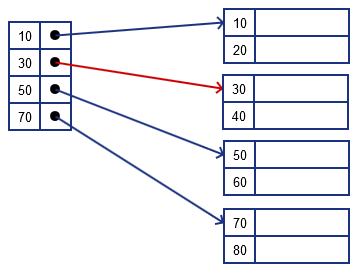suppose we want to delete $k = 40$

• we locate the record, remove it and leave a tombstone there
•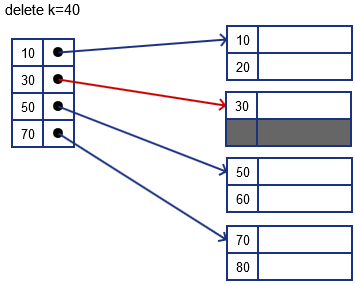if we want to delete $k = 30$

• locate the record, delete it and leave a tombstone there
• but we want to keep it sequential and continuous: so we move 40 up
•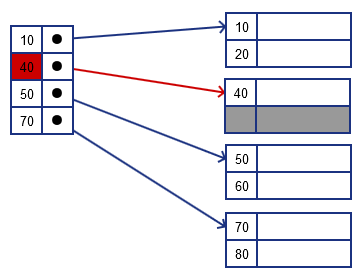• also note that we need to update the index as well: not it needs to point to 40 instead of 30

what if we want to delete both $k = 30$ and $k = 40$?

• locate the records and delete both
• the whole block is no longer needed - we remove the pointers to it along with corresponding index record
• so we remove 30 from the index and move 50 and 70 up
•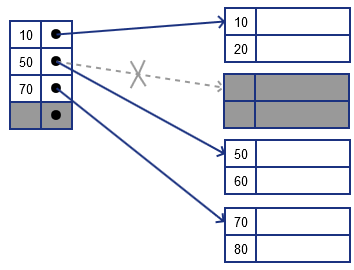### Insertion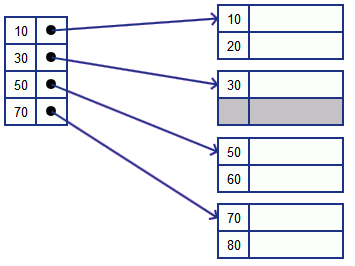insert key 34

• we first locate where we insert
• lucky case: some room in the block, just add the record there

insert key 15

• this time no room in the block where we want to insert it
• there are two options
• Immediate Reorganization
• Overflow Blocks

Immediate Reorganization

• re-distribution data
•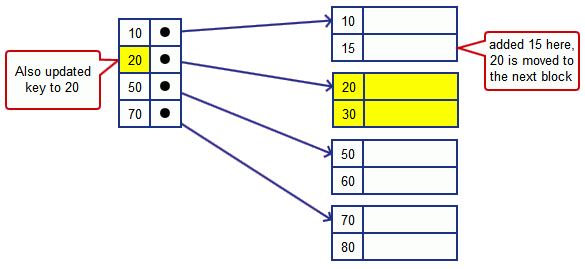• we try to push the data down
• in this case 20 is moved to the next block
• note that we also have to update the index key that points to the second block since its first key got updated
• worst case: we will move all the data blocks
• variant: insert a new block and update the index

Overflow Blocks

• we create a new block and create a pointer to it from
• it will be reorganized later
•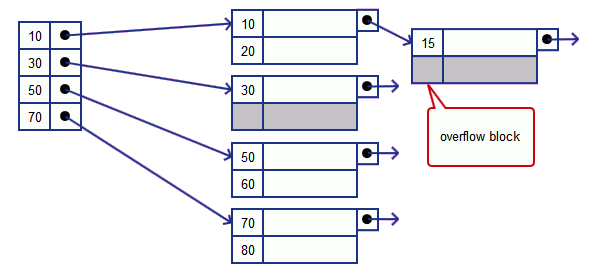• it may degenerate to a linked list
• may have to traverse the whole chain only to find out that the value is not there
• i.e. back to linear search

## Duplicate Keys

Suppose we have duplicate keys in our database. How to build index?

### Option 1

There could be some problems we build it same way as without assuming duplicate keys

•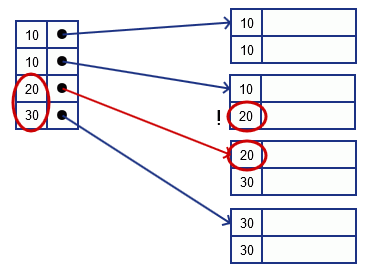• careful with looking for 20 or 30!
• if we follow the pointer for 20, we'll loose the previous record for 20
• so in this case will need to also load the previous block to check if it contains something

### Option 2

We may point to previous values, so we know the range

•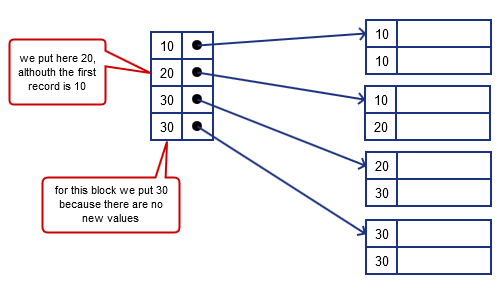• so in the index we indicate the first new (not seen previously) key from block